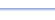Program: POLYGON

POLYGON

• Finds the sum of the interior angles of a convex polygon, given the number of sides.
• If the polygon is regular, it finds the measure of each interior and each exterior angle.

Useful for:

• SAT
• ACT
• Geometry
• Trigonometry
• Precalculus

See full list of programs

Example

Q:

a. Find the sum of the interior angles of a convex decagon.

b. Find the measure of an interior angle and an exterior angle of a regular decagon.

Solution:  Run POLYGON.  How?

Enter 10 for the
number of sides
because a decagon
has 10 sides.

The program reports:

Press ENTER for more
information.

The program reports:

Interpretation:
a. The sum of the interior angles is 1440.
b. The interior angle is 144.  The exterior angle is 36.  (Note that this info is ONLY if it is a regular polygon.)----------------->